<![CDATA[Math Is Fun Forum / Euler Avenue]]> 2022-01-15T14:29:11Z FluxBB https://www.mathisfunforum.com/index.php <![CDATA[Some special numbers]]> 44) Permutable prime

A permutable prime, also known as anagrammatic prime, is a prime number which, in a given base, can have its digits' positions switched through any permutation and still be a prime number. H. E. Richert, who is supposedly the first to study these primes, called them permutable primes, but later they were also called absolute primes.

In base 10, all the permutable primes with fewer than 49,081 digits are known

Of the above, there are 16 unique permutation sets, with smallest elements

Note

is a repunit, a number consisting only of n ones (in base 10). Any repunit prime is a permutable prime with the above definition, but some definitions require at least two distinct digits.

All permutable primes of two or more digits are composed from the digits 1, 3, 7, 9, because no prime number except 2 is even, and no prime number besides 5 is divisible by 5. It is proven that no permutable prime exists which contains three different of the four digits 1, 3, 7, 9, as well as that there exists no permutable prime composed of two or more of each of two digits selected from 1, 3, 7, 9.

There is no n-digit permutable prime for

which is not a repunit. It is conjectured that there are no non-repunit permutable primes other than those listed above.

]]>
https://www.mathisfunforum.com/profile.php?id=682 2022-01-15T14:29:11Z https://www.mathisfunforum.com/viewtopic.php?id=25786&action=new
<![CDATA[Euler's polyhedron formula]]>Euler's polyhedron formula

Let's begin by introducing the protagonist of this story - Euler's formula:

V - E + F = 2.

Simple though it may look, this little formula encapsulates a fundamental property of those three-dimensional solids we call polyhedra, which have fascinated mathematicians for over 4000 years. Actually I can go further and say that Euler's formula tells us something very deep about shape and space. The formula bears the name of the famous Swiss mathematician Leonhard Euler (1707 - 1783), who would have celebrated his 300th birthday this year.

What is a polyhedron?

Before we examine what Euler's formula tells us, let's look at polyhedra in a bit more detail. A polyhedron is a solid object whose surface is made up of a number of flat faces which themselves are bordered by straight lines. Each face is in fact a polygon, a closed shape in the flat 2-dimensional plane made up of points joined by straight lines.

Before we examine what Euler's formula tells us, let's look at polyhedra in a bit more detail. A polyhedron is a solid object whose surface is made up of a number of flat faces which themselves are bordered by straight lines. Each face is in fact a polygon, a closed shape in the flat 2-dimensional plane made up of points joined by straight lines.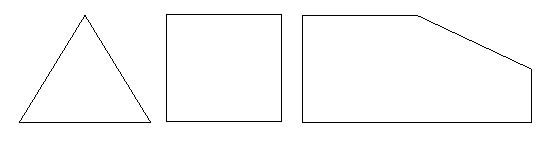A polyhedron is what you get when you move one dimension up. It is a closed, solid object whose surface is made up of a number of polygonal faces. We call the sides of these faces edges — two faces meet along each one of these edges. We call the corners of the faces vertices, so that any vertex lies on at least three different faces. To illustrate this, here are two examples of well-known polyhedra.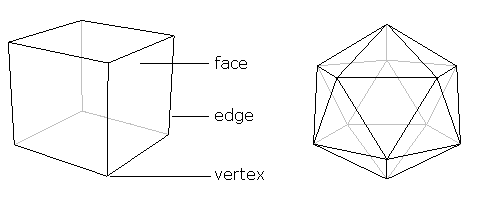A polyhedron consists of just one piece. It cannot, for example, be made up of two (or more) basically separate parts joined by only an edge or a vertex. This means that neither of the following objects is a true polyhedron.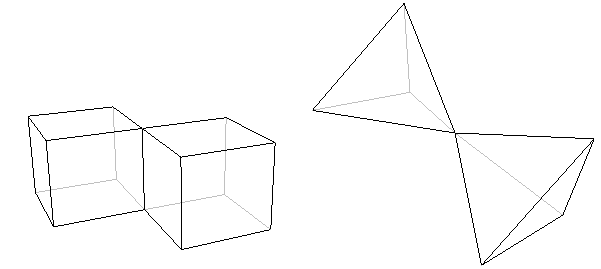What does the formula tell us?

We're now ready to see what Euler's formula tells us about polyhedra. Look at a polyhedron, for example the cube or the icosahedron above, count the number of vertices it has, and call this number V. The cube, for example, has 8 vertices, so V = 8. Next, count the number of edges the polyhedron has, and call this number E. The cube has 12 edges, so in the case of the cube E = 12. Finally, count the number of faces and call it F. In the case of the cube, F = 6. Now Euler's formula tells us that

V - E + F = 2;
or, in words: the number of vertices, minus the number of edges, plus the number of faces, is equal to two.

In the case of the cube, we've already seen that V = 8, E = 12 and F = 6. So,

V - E + F = 8 - 12 + 6 = 14 - 12 = 2
which is what Euler's formula tells us it should be. If we now look at the icosahedron, we find that V = 12, E = 30 and F = 20. Now,

V - E + F = 12 - 30 + 20 = 32 - 30 = 2,
as we expected.

Euler's formula is true for the cube and the icosahedron. It turns out, rather beautifully, that it is true for pretty much every polyhedron. The only polyhedra for which it doesn't work are those that have holes running through them like the one shown in the figure below.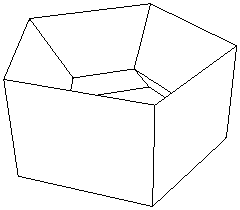These polyhedra are called non-simple, in contrast to the ones that don't have holes, which are called simple. Non-simple polyhedra might not be the first to spring to mind, but there are many of them out there, and we can't get away from the fact that Euler's Formula doesn't work for any of them. However, even this awkward fact has become part of a whole new theory about space and shape.

The power of Euler's formula

Whenever mathematicians hit on an invariant feature, a property that is true for a whole class of objects, they know that they're onto something good. They use it to investigate what properties an individual object can have and to identify properties that all of them must have. Euler's formula can tell us, for example, that there is no simple polyhedron with exactly seven edges. You don't have to sit down with cardboard, scissors and glue to find this out — the formula is all you need. The argument showing that there is no seven-edged polyhedron is quite simple, so have a look at it if you're interested.

Using Euler's formula in a similar way we can discover that there is no simple polyhedron with ten faces and seventeen vertices. The prism shown below, which has an octagon as its base, does have ten faces, but the number of vertices here is sixteen. The pyramid, which has a 9-sided base, also has ten faces, but has ten vertices. But Euler's formula tells us that no simple polyhedron has exactly ten faces and seventeen vertices.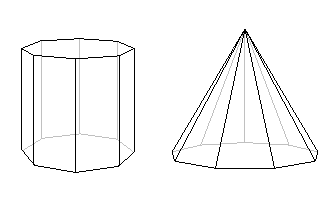It's considerations like these that lead us to what's probably the most beautiful discovery of all. It involves the Platonic Solids, a well-known class of polyhedra named after the ancient Greek philosopher Plato, in whose writings they first appeared.Although their symmetric elegance is immediately apparent when you look at the examples above, it's not actually that easy to pin it down in words. It turns out that it is described by two features. The first is that Platonic solids have no spikes or dips in them, so their shape is nice and rounded. In other words, this means that whenever you choose two points in a Platonic solid and draw a straight line between them, this piece of straight line will be completely contained within the solid — a Platonic solid is what is called convex. The second feature, called regularity, is that all the solid's faces are regular polygons with exactly the same number of sides, and that the same number of edges come out of each vertex of the solid.

The cube is regular, since all its faces are squares and exactly three edges come out of each vertex. You can verify for yourself that the tetrahedron, the octahedron, the icosahedron and the dodecahedron are also regular.

Now, you might wonder how many different Platonic Solids there are. Ever since the discovery of the cube and tetrahedron, mathematicians were so attracted by the elegance and symmetry of the Platonic Solids that they searched for more, and attempted to list all of them. This is where Euler's formula comes in. You can use it to find all the possibilities for the numbers of faces, edges and vertices of a regular polyhedron.What you will discover is that there are in fact only five different regular convex polyhedra! This is very surprising; after all, there is no limit to the number of different regular polygons, so why should we expect a limit here? The five Platonic Solids are the tetrahedron, the cube, the octahedron, the icosahedron and the dodecahedron shown above.

The proof

Playing around with various simple polyhedra will show you that Euler's formula always holds true. But if you're a mathematician, this isn't enough. You'll want a proof, a water-tight logical argument that shows you that it really works for all polyhedra, including the ones you'll never have the time to check.

Despite the formula's name, it wasn't in fact Euler who came up with the first complete proof. Its history is complex, spanning 200 years and involving some of the greatest names in maths, including René Descartes (1596 - 1650), Euler himself, Adrien-Marie Legendre (1752 - 1833) and Augustin-Louis Cauchy (1789 - 1857).

It's interesting to note that all these mathematicians used very different approaches to prove the formula, each striking in its ingenuity and insight. It's Cauchy's proof, though, that I'd like to give you a flavour of here. His method consists of several stages and steps. The first stage involves constructing what is called a network.

]]>
https://www.mathisfunforum.com/profile.php?id=682 2021-12-08T09:38:34Z https://www.mathisfunforum.com/viewtopic.php?id=26850&action=new
<![CDATA[Some Special Numbers - Part 2.]]> a) 11 is the fifth prime and first palindromic multi-digit number in base 10.

b) 12 is the first sublime number.

In number theory, a sublime number is a positive integer which has a perfect number of positive factors (including itself), and whose positive factors add up to another perfect number.

The number 12, for example, is a sublime number. It has a perfect number of positive factors (6): 1, 2, 3, 4, 6, and 12, and the sum of these is again a perfect number: 1 + 2 + 3 + 4 + 6 + 12 = 28.

There are only two known sublime numbers: 12 and

. The second of these has 76 decimal digits:

6,086,555,670,238,378,989,670,371,734,243,169,622,657,830,773,351,885,970,528,324,860,512,791,691,264.

c) We know 6 is the first perfect number : Sum of the factors whose proper factors sum to the number itself.

(1 + 2 + 3 = 6).

28 is the second perfect number.

496 is the third perfect number.

8128 is the fourth perfect number.

d) 17 is the sum of the first 4 prime numbers, and the only prime which is the sum of 4 consecutive primes.

e) 25 is the first centered square number besides 1 that is also a square number.

In elementary number theory, a centered square number is a centered figurate number that gives the number of dots in a square with a dot in the center and all other dots surrounding the center dot in successive square layers. That is, each centered square number equals the number of dots within a given city block distance of the center dot on a regular square lattice. While centered square numbers, like figurate numbers in general, have few if any direct practical applications, they are sometimes studied in recreational mathematics for their elegant geometric and arithmetic properties.

f) 30 is the smallest sphenic number.

In number theory, a sphenic number is a positive integer that is the product of three distinct prime numbers.

Definition : A sphenic number is a product pqr where p, q, and r are three distinct prime numbers. In other words, the sphenic numbers are the square-free 3-almost primes.

Examples : The smallest sphenic number is 30 = 2 × 3 × 5, the product of the smallest three primes. The first few sphenic numbers are

30, 42, 66, 70, 78, 102, 105, 110, 114, 130, 138, 154, 165, ...

g) 142857 is the smallest base 10 cyclic number.

A cyclic number is an integer in which cyclic permutations of the digits are successive integer multiples of the number. The most widely known is the six-digit number 142857, whose first six integer multiples are

142857 × 1 = 142857
142857 × 2 = 285714
142857 × 3 = 428571
142857 × 4 = 571428
142857 × 5 = 714285
142857 × 6 = 857142.

h) 9814072356 is the largest perfect power that contains no repeated digits in base ten.

i) Pandigital number: In mathematics, a pandigital number is an integer that in a given base has among its significant digits each digit used in the base at least once. For example, 1234567890 is a pandigital number in base 10. The first few pandigital base 10 numbers are given by :

1023456789, 1023456798, 1023456879, 1023456897, 1023456978, 1023456987, 1023457689.

]]>
https://www.mathisfunforum.com/profile.php?id=682 2021-11-23T08:25:09Z https://www.mathisfunforum.com/viewtopic.php?id=26799&action=new
<![CDATA[x ° y = y ° x]]> Thank you zetafunc, I do appreciate the various solutions in case as they may come in handy

To be sure I understand I'm going to apply what you said first to the related case of

Taking logs of both sides we get:

Rearranging:

If we now make the y=x^t substitution:

And since y=x^t:

We now have a way to generate solutions. When I try to use this method to find those more complicated answers I began listing earlier, however, I still don't know how, other than by graphing it (which is very difficult for negative answers). For example, graphically it seems there must be some t the real part of which is around -2.56 for which

, yielding the solution to the original problem y=2, x=-0.767... . Is this what happens when you consider t over all the complex numbers? I can see now the solution space for positive t is rather simple (for both equations), but it's still tricky to assess when t is negative. I suppose I am still uninformed, and will come back in a little bit.]]>
https://www.mathisfunforum.com/profile.php?id=212090 2021-10-23T22:11:23Z https://www.mathisfunforum.com/viewtopic.php?id=26703&action=new
<![CDATA[Self learning maths]]> You are in the right place. You can interact with many math enthusiasts! You can shared your knowledge with youngsters and teenagers.

]]>
https://www.mathisfunforum.com/profile.php?id=682 2021-09-07T00:36:05Z https://www.mathisfunforum.com/viewtopic.php?id=25548&action=new
<![CDATA[Knuth's up-arrow notation]]> In mathematics, Knuth's up-arrow notation is a method of notation for very large integers, introduced by Donald Knuth in 1976.

In his 1947 paper, R. L. Goodstein introduced the specific sequence of operations that are now called hyperoperations. Goodstein also suggested the Greek names tetration, pentation, etc., for the extended operations beyond exponentiation. The sequence starts with a unary operation (the successor function with n = 0), and continues with the binary operations of addition (n = 1), multiplication (n = 2), exponentiation (n = 3), tetration (n = 4), pentation (n = 5), etc.

Various notations have been used to represent hyperoperations. One such notation is

. Another notation is
, an infix notation which is convenient for ASCII. The notation
is known as 'square bracket notation'.

Knuth's up-arrow notation

is an alternative notation. It is obtained by replacing
in the square bracket notation by
arrows.

For example:

the single arrow

represents exponentiation (iterated multiplication)

the double arrow
represents tetration (iterated exponentiation)

the triple arrow
represents pentation (iterated tetration)

The general definition of the up-arrow notation is as follows (for
:

Here,
stands for n arrows, so for example

.

]]>
https://www.mathisfunforum.com/profile.php?id=682 2021-06-11T04:46:06Z https://www.mathisfunforum.com/viewtopic.php?id=26378&action=new
<![CDATA[e^x]]> As a teacher with many years of experience I know that the starting point in any lesson is to check that the pupils have understood the previous lesson.  From my point of view, there is no point spending my time adding the next steps if you haven't got a good grasp of what I have said so far.  I'm surprised you feel ready for the next step so soon after getting the link to the thread.

So I want something from you first:

Using a graph plotter, try a = 4 then 5 then 1 then 1/2  then 1/3.

Comment on what you are observing in these cases.  Make three conclusions about how the value of a affects the graph.

Then use the series expansion for e^x with x = 1 to compute e to 8 decimal places .

Post the steps in your working and your final result.  Pay particular attention to explaining how you know your answer is accurate to 8 dp (without just comparing with a published result).

Bob

]]>
https://www.mathisfunforum.com/profile.php?id=67694 2021-05-15T19:45:24Z https://www.mathisfunforum.com/viewtopic.php?id=25550&action=new
<![CDATA[Would certain 3D objects be viewed as impossible in 4D?]]> Bob Bundy,

the impossible cube and the hypercube are different examples, right?

]]>
https://www.mathisfunforum.com/profile.php?id=95904 2020-07-04T00:36:15Z https://www.mathisfunforum.com/viewtopic.php?id=25753&action=new
<![CDATA[How would I write an if statement in an equation?]]> Actually, piecewise functions are really just notation. Do you know how functions are usually viewed in modern mathematics?

]]>
https://www.mathisfunforum.com/profile.php?id=95904 2020-07-01T00:56:02Z https://www.mathisfunforum.com/viewtopic.php?id=25745&action=new
<![CDATA[Imaginary number]]> Hi,

If r and θ are modulus and amplitude of a complex number,
then z=r(cosθ+isinθ).

Argument of Z and Amplitude of Z mean the same thing and are used interchangeably when we talk about complex numbers. When we plot the point of complex number on graph, and join it to the origin, the angle it makes with the x-axis is the argument or amplitude of complex number Z.

Amplitude.

Amplitude(or Argument) of a complex number:

Let z=x+iy  where x,y  are real,

and
; then the value of
for which the equations:

…(1) and

…(2)

are simultaneously satisfied is called the Argument(or Amplitude) of z  and is denoted by Arg z (or,

) .

Clearly, equations (1) and (2) are satisfied for infinite values of \theta ; any of these values of

is the value of Amp z . However, the unique value of
lying in the interval
and satisfying equations (1) and (2) is called the principal value of Arg z  and we denote this principal value by arg z  or amp z .Unless otherwise mentioned, by argument of a complex number we mean its principal value.

Since,

and
(where n=any integer), it follows that,

where
.

]]>
https://www.mathisfunforum.com/profile.php?id=682 2020-04-14T01:51:50Z https://www.mathisfunforum.com/viewtopic.php?id=25576&action=new
<![CDATA[Limits]]> hi 666 bro

Thanks for that link to U Tube.  Now I understand your difficulty.

(1) Make sure your mic volume is set correctly.  Even on 100% volume I could barely hear the speaker.
(2) Plan your 'board' layout in advance so you don't have wobbly lines and have to rub out bits because they won't fit.
(3) Use different colours but only those that have a decent contrast with the black background.  Yellow is excellent; purple is very poor and barely readable.  Have printed text not handwritten notes so that we don't have to struggle reading your writing.

Sorry 666 bro but I felt I needed to get that off my chest.  No wonder you are struggling with this.  It's great that the Academy do this for free but they could learn a lot from MIF.  I suggest you look instead at this page:

https://www.mathsisfun.com/calculus/limits-formal.html

Compare the two and you'll see why I think MIF is such a brilliant resource.

Hope that helps,

Bob

]]>
https://www.mathisfunforum.com/profile.php?id=67694 2020-03-29T12:54:10Z https://www.mathisfunforum.com/viewtopic.php?id=25566&action=new
<![CDATA[Sequences]]> Did you mean order in probability?

]]>
https://www.mathisfunforum.com/profile.php?id=220123 2020-03-28T02:32:58Z https://www.mathisfunforum.com/viewtopic.php?id=25559&action=new
<![CDATA[Binomial theorem]]> Hi 666 bro,

It's used in statistics for the binomial distribution and to prove the differentiation rule for powers of x.

It crops up in Pascal's triangle and everything that follows from that.

There's a way to calculate the constant 'e' that uses the theorem.

I expect others could add to this list.

Have a look at this:

https://www.mathsisfun.com/data/quincunx.html

Bob

]]>
https://www.mathisfunforum.com/profile.php?id=67694 2020-03-18T10:01:43Z https://www.mathisfunforum.com/viewtopic.php?id=25543&action=new
<![CDATA[Publishing for Amateur Mathematicians]]> Mr Reuele
You need not get discouraged. You can get your paper published if your research has some substance in it. Better inspect some research papers already published and try to write in that way as far as style and formatting is concerned. Every journal has an ‘archive’ where one can find earlier published papers. Also go through ‘guidelines for authors’ and follow these. Since you have no affiliation with any university and it is quite probable you have researched in a way different from others, your paper may be taken seriously only if you have done something really remarkable. It is also probable your paper may have to pass through preliminary scrutiny. It is correct, most mathematics journals accept papers in LaTex but there are some journals that accepts papers in Microsoft word using math-type or equation editor for writing mathematical equations, symbols likewise. Please do not lose heart, you can learn how to write in ms word using math type or equation editor. Of course, you will have to forgo those journals which ask paper in LaTex style. I do not hold degree in mathematics, that is not a handicap. It is proving an asset to me because my mind is not biased the way other mathematicians have done their work. My approach is independent and altogether different from peers. My first research paper has been published and is available at http://www.ceser.in/ceserp/index.php/IJEFT/article/view/6203 If you have interest in the subject, you may go through. Another paper has been accepted and is likely to be published next year and some are under review. Last, where there is a will there a way. Wishing you all the best!

]]>
https://www.mathisfunforum.com/profile.php?id=220789 2019-11-06T12:42:31Z https://www.mathisfunforum.com/viewtopic.php?id=21594&action=new
<![CDATA[What had you learned today that you found interesting?]]> Today I learned the word thigmomorphogenesis, which is the way plants grow in response to touch.

]]>
https://www.mathisfunforum.com/profile.php?id=220188 2019-07-02T19:41:37Z https://www.mathisfunforum.com/viewtopic.php?id=21753&action=new